# Ragged tensors

Stay organized with collections Save and categorize content based on your preferences.

API Documentation: `tf.RaggedTensor` `tf.ragged`

## Setup

``````!pip install --pre -U tensorflow
import math
import tensorflow as tf
``````

## Overview

Your data comes in many shapes; your tensors should too. Ragged tensors are the TensorFlow equivalent of nested variable-length lists. They make it easy to store and process data with non-uniform shapes, including:

• Variable-length features, such as the set of actors in a movie.
• Batches of variable-length sequential inputs, such as sentences or video clips.
• Hierarchical inputs, such as text documents that are subdivided into sections, paragraphs, sentences, and words.
• Individual fields in structured inputs, such as protocol buffers.

### What you can do with a ragged tensor

Ragged tensors are supported by more than a hundred TensorFlow operations, including math operations (such as `tf.add` and `tf.reduce_mean`), array operations (such as `tf.concat` and `tf.tile`), string manipulation ops (such as `tf.strings.substr`), control flow operations (such as `tf.while_loop` and `tf.map_fn`), and many others:

``````digits = tf.ragged.constant([[3, 1, 4, 1], [], [5, 9, 2], , []])
words = tf.ragged.constant([["So", "long"], ["thanks", "for", "all", "the", "fish"]])
print(tf.reduce_mean(digits, axis=1))
print(tf.concat([digits, [[5, 3]]], axis=0))
print(tf.tile(digits, [1, 2]))
print(tf.strings.substr(words, 0, 2))
print(tf.map_fn(tf.math.square, digits))
``````
```<tf.RaggedTensor [[6, 4, 7, 4], [], [8, 12, 5], , []]>
tf.Tensor([2.25              nan 5.33333333 6.                nan], shape=(5,), dtype=float64)
<tf.RaggedTensor [[3, 1, 4, 1], [], [5, 9, 2], , [], [5, 3]]>
<tf.RaggedTensor [[3, 1, 4, 1, 3, 1, 4, 1], [], [5, 9, 2, 5, 9, 2], [6, 6], []]>
<tf.RaggedTensor [[b'So', b'lo'], [b'th', b'fo', b'al', b'th', b'fi']]>
<tf.RaggedTensor [[9, 1, 16, 1], [], [25, 81, 4], , []]>
```

There are also a number of methods and operations that are specific to ragged tensors, including factory methods, conversion methods, and value-mapping operations. For a list of supported ops, see the `tf.ragged` package documentation.

Ragged tensors are supported by many TensorFlow APIs, including Keras, Datasets, tf.function, SavedModels, and tf.Example. For more information, check the section on TensorFlow APIs below.

As with normal tensors, you can use Python-style indexing to access specific slices of a ragged tensor. For more information, refer to the section on Indexing below.

``````print(digits)       # First row
``````
```tf.Tensor([3 1 4 1], shape=(4,), dtype=int32)
```
``````print(digits[:, :2])   # First two values in each row.
``````
```<tf.RaggedTensor [[3, 1], [], [5, 9], , []]>
```
``````print(digits[:, -2:])  # Last two values in each row.
``````
```<tf.RaggedTensor [[4, 1], [], [9, 2], , []]>
```

And just like normal tensors, you can use Python arithmetic and comparison operators to perform elementwise operations. For more information, check the section on Overloaded operators below.

``````print(digits + 3)
``````
```<tf.RaggedTensor [[6, 4, 7, 4], [], [8, 12, 5], , []]>
```
``````print(digits + tf.ragged.constant([[1, 2, 3, 4], [], [5, 6, 7], , []]))
``````
```<tf.RaggedTensor [[4, 3, 7, 5], [], [10, 15, 9], , []]>
```

If you need to perform an elementwise transformation to the values of a `RaggedTensor`, you can use `tf.ragged.map_flat_values`, which takes a function plus one or more arguments, and applies the function to transform the `RaggedTensor`'s values.

``````times_two_plus_one = lambda x: x * 2 + 1
print(tf.ragged.map_flat_values(times_two_plus_one, digits))
``````
```<tf.RaggedTensor [[7, 3, 9, 3], [], [11, 19, 5], , []]>
```

Ragged tensors can be converted to nested Python `list`s and NumPy `array`s:

``````digits.to_list()
``````
```[[3, 1, 4, 1], [], [5, 9, 2], , []]
```
``````digits.numpy()
``````
```array([array([3, 1, 4, 1], dtype=int32), array([], dtype=int32),
array([5, 9, 2], dtype=int32), array(, dtype=int32),
array([], dtype=int32)], dtype=object)
```

### Constructing a ragged tensor

The simplest way to construct a ragged tensor is using `tf.ragged.constant`, which builds the `RaggedTensor` corresponding to a given nested Python `list` or NumPy `array`:

``````sentences = tf.ragged.constant([
["Let's", "build", "some", "ragged", "tensors", "!"],
["We", "can", "use", "tf.ragged.constant", "."]])
print(sentences)
``````
```<tf.RaggedTensor [[b"Let's", b'build', b'some', b'ragged', b'tensors', b'!'],
[b'We', b'can', b'use', b'tf.ragged.constant', b'.']]>
```
``````paragraphs = tf.ragged.constant([
[['I', 'have', 'a', 'cat'], ['His', 'name', 'is', 'Mat']],
[['Do', 'you', 'want', 'to', 'come', 'visit'], ["I'm", 'free', 'tomorrow']],
])
print(paragraphs)
``````
```<tf.RaggedTensor [[[b'I', b'have', b'a', b'cat'], [b'His', b'name', b'is', b'Mat']],
[[b'Do', b'you', b'want', b'to', b'come', b'visit'],
[b"I'm", b'free', b'tomorrow']]]>
```

Ragged tensors can also be constructed by pairing flat values tensors with row-partitioning tensors indicating how those values should be divided into rows, using factory classmethods such as `tf.RaggedTensor.from_value_rowids`, `tf.RaggedTensor.from_row_lengths`, and `tf.RaggedTensor.from_row_splits`.

#### `tf.RaggedTensor.from_value_rowids`

If you know which row each value belongs to, then you can build a `RaggedTensor` using a `value_rowids` row-partitioning tensor: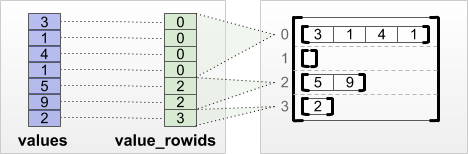``````print(tf.RaggedTensor.from_value_rowids(
values=[3, 1, 4, 1, 5, 9, 2],
value_rowids=[0, 0, 0, 0, 2, 2, 3]))
``````
```<tf.RaggedTensor [[3, 1, 4, 1], [], [5, 9], ]>
```

#### `tf.RaggedTensor.from_row_lengths`

If you know how long each row is, then you can use a `row_lengths` row-partitioning tensor: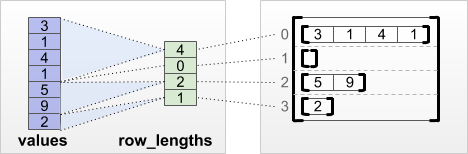``````print(tf.RaggedTensor.from_row_lengths(
values=[3, 1, 4, 1, 5, 9, 2],
row_lengths=[4, 0, 2, 1]))
``````
```<tf.RaggedTensor [[3, 1, 4, 1], [], [5, 9], ]>
```

#### `tf.RaggedTensor.from_row_splits`

If you know the index where each row starts and ends, then you can use a `row_splits` row-partitioning tensor: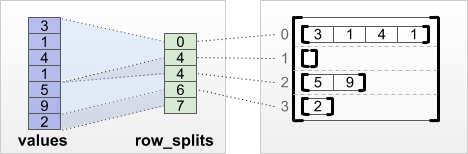``````print(tf.RaggedTensor.from_row_splits(
values=[3, 1, 4, 1, 5, 9, 2],
row_splits=[0, 4, 4, 6, 7]))
``````
```<tf.RaggedTensor [[3, 1, 4, 1], [], [5, 9], ]>
```

See the `tf.RaggedTensor` class documentation for a full list of factory methods.

### What you can store in a ragged tensor

As with normal `Tensor`s, the values in a `RaggedTensor` must all have the same type; and the values must all be at the same nesting depth (the rank of the tensor):

``````print(tf.ragged.constant([["Hi"], ["How", "are", "you"]]))  # ok: type=string, rank=2
``````
```<tf.RaggedTensor [[b'Hi'], [b'How', b'are', b'you']]>
```
``````print(tf.ragged.constant([[[1, 2], ], [[4, 5]]]))        # ok: type=int32, rank=3
``````
```<tf.RaggedTensor [[[1, 2], ], [[4, 5]]]>
```
``````try:
tf.ragged.constant([["one", "two"], [3, 4]])              # bad: multiple types
except ValueError as exception:
print(exception)
``````
```Can't convert Python sequence with mixed types to Tensor.
```
``````try:
tf.ragged.constant(["A", ["B", "C"]])                     # bad: multiple nesting depths
except ValueError as exception:
print(exception)
``````
```all scalar values must have the same nesting depth
```

## Example use case

The following example demonstrates how `RaggedTensor`s can be used to construct and combine unigram and bigram embeddings for a batch of variable-length queries, using special markers for the beginning and end of each sentence. For more details on the ops used in this example, check the `tf.ragged` package documentation.

``````queries = tf.ragged.constant([['Who', 'is', 'Dan', 'Smith'],
['Pause'],
['Will', 'it', 'rain', 'later', 'today']])

# Create an embedding table.
num_buckets = 1024
embedding_size = 4
embedding_table = tf.Variable(
tf.random.truncated_normal([num_buckets, embedding_size],
stddev=1.0 / math.sqrt(embedding_size)))

# Look up the embedding for each word.
word_buckets = tf.strings.to_hash_bucket_fast(queries, num_buckets)
word_embeddings = tf.nn.embedding_lookup(embedding_table, word_buckets)     # ①

# Add markers to the beginning and end of each sentence.
marker = tf.fill([queries.nrows(), 1], '#')
padded = tf.concat([marker, queries, marker], axis=1)                       # ②

# Build word bigrams and look up embeddings.

bigram_buckets = tf.strings.to_hash_bucket_fast(bigrams, num_buckets)
bigram_embeddings = tf.nn.embedding_lookup(embedding_table, bigram_buckets) # ④

# Find the average embedding for each sentence
all_embeddings = tf.concat([word_embeddings, bigram_embeddings], axis=1)    # ⑤
avg_embedding = tf.reduce_mean(all_embeddings, axis=1)                      # ⑥
print(avg_embedding)
``````
```tf.Tensor(
[[-0.0955641  -0.01629535  0.07149196  0.13513806]
[ 0.41757596  0.2445757   0.06732988 -0.04196669]
[ 0.21912457 -0.17321034  0.00455054  0.23211549]], shape=(3, 4), dtype=float32)
```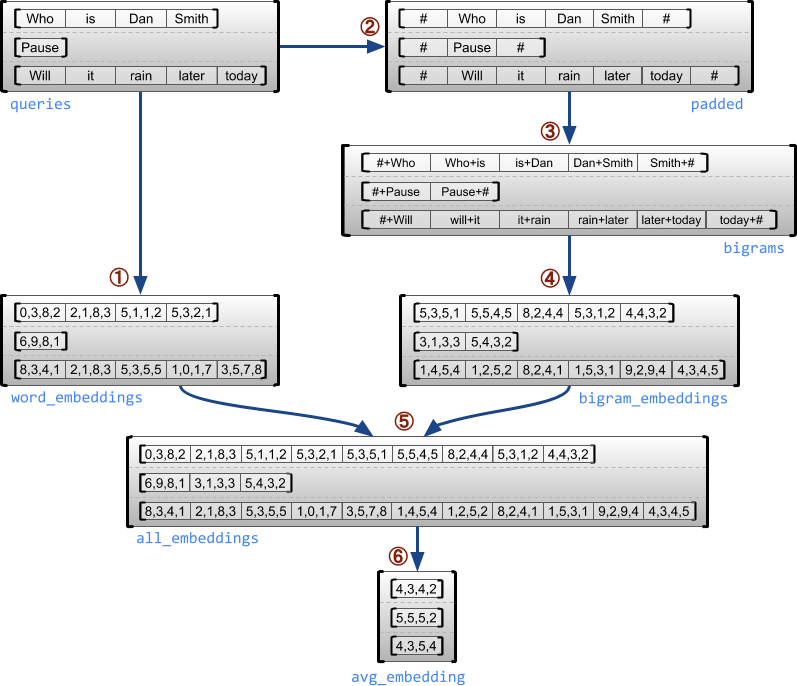## Ragged and uniform dimensions

A ragged dimension is a dimension whose slices may have different lengths. For example, the inner (column) dimension of `rt=[[3, 1, 4, 1], [], [5, 9, 2], , []]` is ragged, since the column slices (`rt[0, :]`, ..., `rt[4, :]`) have different lengths. Dimensions whose slices all have the same length are called uniform dimensions.

The outermost dimension of a ragged tensor is always uniform, since it consists of a single slice (and, therefore, there is no possibility for differing slice lengths). The remaining dimensions may be either ragged or uniform. For example, you may store the word embeddings for each word in a batch of sentences using a ragged tensor with shape `[num_sentences, (num_words), embedding_size]`, where the parentheses around `(num_words)` indicate that the dimension is ragged.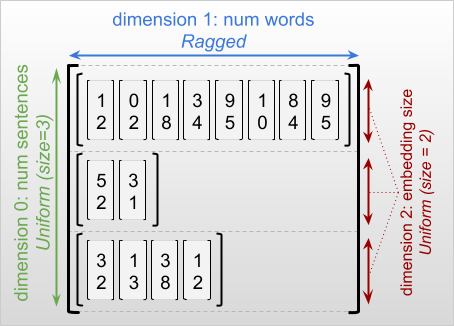Ragged tensors may have multiple ragged dimensions. For example, you could store a batch of structured text documents using a tensor with shape `[num_documents, (num_paragraphs), (num_sentences), (num_words)]` (where again parentheses are used to indicate ragged dimensions).

As with `tf.Tensor`, the rank of a ragged tensor is its total number of dimensions (including both ragged and uniform dimensions). A potentially ragged tensor is a value that might be either a `tf.Tensor` or a `tf.RaggedTensor`.

When describing the shape of a RaggedTensor, ragged dimensions are conventionally indicated by enclosing them in parentheses. For example, as you saw above, the shape of a 3D RaggedTensor that stores word embeddings for each word in a batch of sentences can be written as `[num_sentences, (num_words), embedding_size]`.

The `RaggedTensor.shape` attribute returns a `tf.TensorShape` for a ragged tensor where ragged dimensions have size `None`:

``````tf.ragged.constant([["Hi"], ["How", "are", "you"]]).shape
``````
```TensorShape([2, None])
```

The method `tf.RaggedTensor.bounding_shape` can be used to find a tight bounding shape for a given `RaggedTensor`:

``````print(tf.ragged.constant([["Hi"], ["How", "are", "you"]]).bounding_shape())
``````
```tf.Tensor([2 3], shape=(2,), dtype=int64)
```

## Ragged vs sparse

A ragged tensor should not be thought of as a type of sparse tensor. In particular, sparse tensors are efficient encodings for `tf.Tensor` that model the same data in a compact format; but ragged tensor is an extension to `tf.Tensor` that models an expanded class of data. This difference is crucial when defining operations:

• Applying an op to a sparse or dense tensor should always give the same result.
• Applying an op to a ragged or sparse tensor may give different results.

As an illustrative example, consider how array operations such as `concat`, `stack`, and `tile` are defined for ragged vs. sparse tensors. Concatenating ragged tensors joins each row to form a single row with the combined length:``````ragged_x = tf.ragged.constant([["John"], ["a", "big", "dog"], ["my", "cat"]])
ragged_y = tf.ragged.constant([["fell", "asleep"], ["barked"], ["is", "fuzzy"]])
print(tf.concat([ragged_x, ragged_y], axis=1))
``````
```<tf.RaggedTensor [[b'John', b'fell', b'asleep'], [b'a', b'big', b'dog', b'barked'],
[b'my', b'cat', b'is', b'fuzzy']]>
```

However, concatenating sparse tensors is equivalent to concatenating the corresponding dense tensors, as illustrated by the following example (where Ø indicates missing values):``````sparse_x = ragged_x.to_sparse()
sparse_y = ragged_y.to_sparse()
sparse_result = tf.sparse.concat(sp_inputs=[sparse_x, sparse_y], axis=1)
print(tf.sparse.to_dense(sparse_result, ''))
``````
```tf.Tensor(
[[b'John' b'' b'' b'fell' b'asleep']
[b'a' b'big' b'dog' b'barked' b'']
[b'my' b'cat' b'' b'is' b'fuzzy']], shape=(3, 5), dtype=string)
```

For another example of why this distinction is important, consider the definition of “the mean value of each row” for an op such as `tf.reduce_mean`. For a ragged tensor, the mean value for a row is the sum of the row’s values divided by the row’s width. But for a sparse tensor, the mean value for a row is the sum of the row’s values divided by the sparse tensor’s overall width (which is greater than or equal to the width of the longest row).

## TensorFlow APIs

### Keras

tf.keras is TensorFlow's high-level API for building and training deep learning models. Ragged tensors may be passed as inputs to a Keras model by setting `ragged=True` on `tf.keras.Input` or `tf.keras.layers.InputLayer`. Ragged tensors may also be passed between Keras layers, and returned by Keras models. The following example shows a toy LSTM model that is trained using ragged tensors.

``````# Task: predict whether each sentence is a question or not.
sentences = tf.constant(
['What makes you think she is a witch?',
'She turned me into a newt.',
'A newt?',
'Well, I got better.'])
is_question = tf.constant([True, False, True, False])

# Preprocess the input strings.
hash_buckets = 1000
words = tf.strings.split(sentences, ' ')
hashed_words = tf.strings.to_hash_bucket_fast(words, hash_buckets)

# Build the Keras model.
keras_model = tf.keras.Sequential([
tf.keras.layers.Input(shape=[None], dtype=tf.int64, ragged=True),
tf.keras.layers.Embedding(hash_buckets, 16),
tf.keras.layers.LSTM(32, use_bias=False),
tf.keras.layers.Dense(32),
tf.keras.layers.Activation(tf.nn.relu),
tf.keras.layers.Dense(1)
])

keras_model.compile(loss='binary_crossentropy', optimizer='rmsprop')
keras_model.fit(hashed_words, is_question, epochs=5)
print(keras_model.predict(hashed_words))
``````
```WARNING:tensorflow:Layer lstm will not use cuDNN kernels since it doesn't meet the criteria. It will use a generic GPU kernel as fallback when running on GPU.
Epoch 1/5
1/1 [==============================] - 3s 3s/step - loss: 7.7125
Epoch 2/5
1/1 [==============================] - 0s 17ms/step - loss: 7.7125
Epoch 3/5
1/1 [==============================] - 0s 15ms/step - loss: 7.7125
Epoch 4/5
1/1 [==============================] - 0s 16ms/step - loss: 7.7125
Epoch 5/5
1/1 [==============================] - 0s 15ms/step - loss: 7.7125
1/1 [==============================] - 0s 184ms/step
[[-0.00090485]
[-0.00014753]
[-0.00211005]
[-0.00238474]]
```

### tf.Example

tf.Example is a standard protobuf encoding for TensorFlow data. Data encoded with `tf.Example`s often includes variable-length features. For example, the following code defines a batch of four `tf.Example` messages with different feature lengths:

``````import google.protobuf.text_format as pbtext

def build_tf_example(s):
return pbtext.Merge(s, tf.train.Example()).SerializeToString()

example_batch = [
build_tf_example(r'''
features {
feature {key: "colors" value {bytes_list {value: ["red", "blue"]} } }
feature {key: "lengths" value {int64_list {value: } } } }'''),
build_tf_example(r'''
features {
feature {key: "colors" value {bytes_list {value: ["orange"]} } }
feature {key: "lengths" value {int64_list {value: []} } } }'''),
build_tf_example(r'''
features {
feature {key: "colors" value {bytes_list {value: ["black", "yellow"]} } }
feature {key: "lengths" value {int64_list {value: [1, 3]} } } }'''),
build_tf_example(r'''
features {
feature {key: "colors" value {bytes_list {value: ["green"]} } }
feature {key: "lengths" value {int64_list {value: [3, 5, 2]} } } }''')]
``````

You can parse this encoded data using `tf.io.parse_example`, which takes a tensor of serialized strings and a feature specification dictionary, and returns a dictionary mapping feature names to tensors. To read the variable-length features into ragged tensors, you simply use `tf.io.RaggedFeature` in the feature specification dictionary:

``````feature_specification = {
'colors': tf.io.RaggedFeature(tf.string),
'lengths': tf.io.RaggedFeature(tf.int64),
}
feature_tensors = tf.io.parse_example(example_batch, feature_specification)
for name, value in feature_tensors.items():
print("{}={}".format(name, value))
``````
```colors=<tf.RaggedTensor [[b'red', b'blue'], [b'orange'], [b'black', b'yellow'], [b'green']]>
lengths=<tf.RaggedTensor [, [], [1, 3], [3, 5, 2]]>
```

`tf.io.RaggedFeature` can also be used to read features with multiple ragged dimensions. For details, refer to the API documentation.

### Datasets

tf.data is an API that enables you to build complex input pipelines from simple, reusable pieces. Its core data structure is `tf.data.Dataset`, which represents a sequence of elements, in which each element consists of one or more components.

``````# Helper function used to print datasets in the examples below.
def print_dictionary_dataset(dataset):
for i, element in enumerate(dataset):
print("Element {}:".format(i))
for (feature_name, feature_value) in element.items():
print('{:>14} = {}'.format(feature_name, feature_value))
``````

#### Building Datasets with ragged tensors

Datasets can be built from ragged tensors using the same methods that are used to build them from `tf.Tensor`s or NumPy `array`s, such as `Dataset.from_tensor_slices`:

``````dataset = tf.data.Dataset.from_tensor_slices(feature_tensors)
print_dictionary_dataset(dataset)
``````
```Element 0:
colors = [b'red' b'blue']
lengths = 
Element 1:
colors = [b'orange']
lengths = []
Element 2:
colors = [b'black' b'yellow']
lengths = [1 3]
Element 3:
colors = [b'green']
lengths = [3 5 2]
```

#### Batching and unbatching Datasets with ragged tensors

Datasets with ragged tensors can be batched (which combines n consecutive elements into a single elements) using the `Dataset.batch` method.

``````batched_dataset = dataset.batch(2)
print_dictionary_dataset(batched_dataset)
``````
```Element 0:
colors = <tf.RaggedTensor [[b'red', b'blue'], [b'orange']]>
lengths = <tf.RaggedTensor [, []]>
Element 1:
colors = <tf.RaggedTensor [[b'black', b'yellow'], [b'green']]>
lengths = <tf.RaggedTensor [[1, 3], [3, 5, 2]]>
```

Conversely, a batched dataset can be transformed into a flat dataset using `Dataset.unbatch`.

``````unbatched_dataset = batched_dataset.unbatch()
print_dictionary_dataset(unbatched_dataset)
``````
```Element 0:
colors = [b'red' b'blue']
lengths = 
Element 1:
colors = [b'orange']
lengths = []
Element 2:
colors = [b'black' b'yellow']
lengths = [1 3]
Element 3:
colors = [b'green']
lengths = [3 5 2]
```

#### Batching Datasets with variable-length non-ragged tensors

If you have a Dataset that contains non-ragged tensors, and tensor lengths vary across elements, then you can batch those non-ragged tensors into ragged tensors by applying the `dense_to_ragged_batch` transformation:

``````non_ragged_dataset = tf.data.Dataset.from_tensor_slices([1, 5, 3, 2, 8])
non_ragged_dataset = non_ragged_dataset.map(tf.range)
batched_non_ragged_dataset = non_ragged_dataset.apply(
tf.data.experimental.dense_to_ragged_batch(2))
for element in batched_non_ragged_dataset:
print(element)
``````
```WARNING:tensorflow:From /tmpfs/tmp/ipykernel_188888/1427668168.py:4: dense_to_ragged_batch (from tensorflow.python.data.experimental.ops.batching) is deprecated and will be removed in a future version.
Instructions for updating:
<tf.RaggedTensor [, [0, 1, 2, 3, 4]]>
<tf.RaggedTensor [[0, 1, 2], [0, 1]]>
<tf.RaggedTensor [[0, 1, 2, 3, 4, 5, 6, 7]]>
```

#### Transforming Datasets with ragged tensors

You can also create or transform ragged tensors in Datasets using `Dataset.map`:

``````def transform_lengths(features):
return {
'mean_length': tf.math.reduce_mean(features['lengths']),
'length_ranges': tf.ragged.range(features['lengths'])}
transformed_dataset = dataset.map(transform_lengths)
print_dictionary_dataset(transformed_dataset)
``````
```Element 0:
mean_length = 7
length_ranges = <tf.RaggedTensor [[0, 1, 2, 3, 4, 5, 6]]>
Element 1:
mean_length = 0
length_ranges = <tf.RaggedTensor []>
Element 2:
mean_length = 2
length_ranges = <tf.RaggedTensor [, [0, 1, 2]]>
Element 3:
mean_length = 3
length_ranges = <tf.RaggedTensor [[0, 1, 2], [0, 1, 2, 3, 4], [0, 1]]>
```

### tf.function

tf.function is a decorator that precomputes TensorFlow graphs for Python functions, which can substantially improve the performance of your TensorFlow code. Ragged tensors can be used transparently with `@tf.function`-decorated functions. For example, the following function works with both ragged and non-ragged tensors:

``````@tf.function
def make_palindrome(x, axis):
return tf.concat([x, tf.reverse(x, [axis])], axis)
``````
``````make_palindrome(tf.constant([[1, 2], [3, 4], [5, 6]]), axis=1)
``````
```<tf.Tensor: shape=(3, 4), dtype=int32, numpy=
array([[1, 2, 2, 1],
[3, 4, 4, 3],
[5, 6, 6, 5]], dtype=int32)>
```
``````make_palindrome(tf.ragged.constant([[1, 2], , [4, 5, 6]]), axis=1)
``````
```2023-03-08 04:07:53.738303: W tensorflow/core/grappler/optimizers/loop_optimizer.cc:907] Skipping loop optimization for Merge node with control input: RaggedConcat/assert_equal_1/Assert/AssertGuard/branch_executed/_9
<tf.RaggedTensor [[1, 2, 2, 1], [3, 3], [4, 5, 6, 6, 5, 4]]>
```

If you wish to explicitly specify the `input_signature` for the `tf.function`, then you can do so using `tf.RaggedTensorSpec`.

``````@tf.function(
input_signature=[tf.RaggedTensorSpec(shape=[None, None], dtype=tf.int32)])
def max_and_min(rt):
return (tf.math.reduce_max(rt, axis=-1), tf.math.reduce_min(rt, axis=-1))

max_and_min(tf.ragged.constant([[1, 2], , [4, 5, 6]]))
``````
```(<tf.Tensor: shape=(3,), dtype=int32, numpy=array([2, 3, 6], dtype=int32)>,
<tf.Tensor: shape=(3,), dtype=int32, numpy=array([1, 3, 4], dtype=int32)>)
```

#### Concrete functions

Concrete functions encapsulate individual traced graphs that are built by `tf.function`. Ragged tensors can be used transparently with concrete functions.

``````@tf.function
def increment(x):
return x + 1

rt = tf.ragged.constant([[1, 2], , [4, 5, 6]])
cf = increment.get_concrete_function(rt)
print(cf(rt))
``````
```<tf.RaggedTensor [[2, 3], , [5, 6, 7]]>
```

### SavedModels

A SavedModel is a serialized TensorFlow program, including both weights and computation. It can be built from a Keras model or from a custom model. In either case, ragged tensors can be used transparently with the functions and methods defined by a SavedModel.

#### Example: saving a Keras model

``````import tempfile

keras_module_path = tempfile.mkdtemp()
tf.saved_model.save(keras_model, keras_module_path)
imported_model(hashed_words)
``````
```WARNING:absl:Function `_wrapped_model` contains input name(s) args_0 with unsupported characters which will be renamed to args_0_1 in the SavedModel.
INFO:tensorflow:Assets written to: /tmpfs/tmp/tmpac64uj9x/assets
INFO:tensorflow:Assets written to: /tmpfs/tmp/tmpac64uj9x/assets
<tf.Tensor: shape=(4, 1), dtype=float32, numpy=
array([[-0.00090485],
[-0.00014753],
[-0.00211005],
[-0.00238474]], dtype=float32)>
```

#### Example: saving a custom model

``````class CustomModule(tf.Module):
def __init__(self, variable_value):
super(CustomModule, self).__init__()
self.v = tf.Variable(variable_value)

@tf.function
def grow(self, x):
return x * self.v

module = CustomModule(100.0)

# Before saving a custom model, you must ensure that concrete functions are
# built for each input signature that you will need.
module.grow.get_concrete_function(tf.RaggedTensorSpec(shape=[None, None],
dtype=tf.float32))

custom_module_path = tempfile.mkdtemp()
tf.saved_model.save(module, custom_module_path)
imported_model.grow(tf.ragged.constant([[1.0, 4.0, 3.0], [2.0]]))
``````
```INFO:tensorflow:Assets written to: /tmpfs/tmp/tmpye6einh1/assets
INFO:tensorflow:Assets written to: /tmpfs/tmp/tmpye6einh1/assets
<tf.RaggedTensor [[100.0, 400.0, 300.0], [200.0]]>
```

The `RaggedTensor` class overloads the standard Python arithmetic and comparison operators, making it easy to perform basic elementwise math:

``````x = tf.ragged.constant([[1, 2], , [4, 5, 6]])
y = tf.ragged.constant([[1, 1], , [3, 3, 3]])
print(x + y)
``````
```<tf.RaggedTensor [[2, 3], , [7, 8, 9]]>
```

Since the overloaded operators perform elementwise computations, the inputs to all binary operations must have the same shape or be broadcastable to the same shape. In the simplest broadcasting case, a single scalar is combined elementwise with each value in a ragged tensor:

``````x = tf.ragged.constant([[1, 2], , [4, 5, 6]])
print(x + 3)
``````
```<tf.RaggedTensor [[4, 5], , [7, 8, 9]]>
```

For a discussion of more advanced cases, check the section on Broadcasting.

Ragged tensors overload the same set of operators as normal `Tensor`s: the unary operators `-`, `~`, and `abs()`; and the binary operators `+`, `-`, `*`, `/`, `//`, `%`, `**`, `&`, `|`, `^`, `==`, `<`, `<=`, `>`, and `>=`.

## Indexing

Ragged tensors support Python-style indexing, including multidimensional indexing and slicing. The following examples demonstrate ragged tensor indexing with a 2D and a 3D ragged tensor.

### Indexing examples: 2D ragged tensor

``````queries = tf.ragged.constant(
[['Who', 'is', 'George', 'Washington'],
['What', 'is', 'the', 'weather', 'tomorrow'],
['Goodnight']])
``````
``````print(queries)                   # A single query
``````
```tf.Tensor([b'What' b'is' b'the' b'weather' b'tomorrow'], shape=(5,), dtype=string)
```
``````print(queries[1, 2])                # A single word
``````
```tf.Tensor(b'the', shape=(), dtype=string)
```
``````print(queries[1:])                  # Everything but the first row
``````
```<tf.RaggedTensor [[b'What', b'is', b'the', b'weather', b'tomorrow'], [b'Goodnight']]>
```
``````print(queries[:, :3])               # The first 3 words of each query
``````
```<tf.RaggedTensor [[b'Who', b'is', b'George'], [b'What', b'is', b'the'], [b'Goodnight']]>
```
``````print(queries[:, -2:])              # The last 2 words of each query
``````
```<tf.RaggedTensor [[b'George', b'Washington'], [b'weather', b'tomorrow'], [b'Goodnight']]>
```

### Indexing examples: 3D ragged tensor

``````rt = tf.ragged.constant([[[1, 2, 3], ],
[, [], ],
[],
[[8, 9], ]])
``````
``````print(rt)                        # Second row (2D RaggedTensor)
``````
```<tf.RaggedTensor [, [], ]>
```
``````print(rt[3, 0])                     # First element of fourth row (1D Tensor)
``````
```tf.Tensor([8 9], shape=(2,), dtype=int32)
```
``````print(rt[:, 1:3])                   # Items 1-3 of each row (3D RaggedTensor)
``````
```<tf.RaggedTensor [[], [[], ], [], []]>
```
``````print(rt[:, -1:])                   # Last item of each row (3D RaggedTensor)
``````
```<tf.RaggedTensor [[],

[],

[],

[]]>
```

`RaggedTensor`s support multidimensional indexing and slicing with one restriction: indexing into a ragged dimension is not allowed. This case is problematic because the indicated value may exist in some rows but not others. In such cases, it's not obvious whether you should (1) raise an `IndexError`; (2) use a default value; or (3) skip that value and return a tensor with fewer rows than you started with. Following the guiding principles of Python ("In the face of ambiguity, refuse the temptation to guess"), this operation is currently disallowed.

## Tensor type conversion

The `RaggedTensor` class defines methods that can be used to convert between `RaggedTensor`s and `tf.Tensor`s or `tf.SparseTensors`:

``````ragged_sentences = tf.ragged.constant([
['Hi'], ['Welcome', 'to', 'the', 'fair'], ['Have', 'fun']])
``````
``````# RaggedTensor -> Tensor
print(ragged_sentences.to_tensor(default_value='', shape=[None, 10]))
``````
```tf.Tensor(
[[b'Hi' b'' b'' b'' b'' b'' b'' b'' b'' b'']
[b'Welcome' b'to' b'the' b'fair' b'' b'' b'' b'' b'' b'']
[b'Have' b'fun' b'' b'' b'' b'' b'' b'' b'' b'']], shape=(3, 10), dtype=string)
```
``````# Tensor -> RaggedTensor
x = [[1, 3, -1, -1], [2, -1, -1, -1], [4, 5, 8, 9]]
``````
```<tf.RaggedTensor [[1, 3], , [4, 5, 8, 9]]>
```
``````#RaggedTensor -> SparseTensor
print(ragged_sentences.to_sparse())
``````
```SparseTensor(indices=tf.Tensor(
[[0 0]
[1 0]
[1 1]
[1 2]
[1 3]
[2 0]
[2 1]], shape=(7, 2), dtype=int64), values=tf.Tensor([b'Hi' b'Welcome' b'to' b'the' b'fair' b'Have' b'fun'], shape=(7,), dtype=string), dense_shape=tf.Tensor([3 4], shape=(2,), dtype=int64))
```
``````# SparseTensor -> RaggedTensor
st = tf.SparseTensor(indices=[[0, 0], [2, 0], [2, 1]],
values=['a', 'b', 'c'],
dense_shape=[3, 3])
print(tf.RaggedTensor.from_sparse(st))
``````
```<tf.RaggedTensor [[b'a'], [], [b'b', b'c']]>
```

## Evaluating ragged tensors

To access the values in a ragged tensor, you can:

1. Use `tf.RaggedTensor.to_list` to convert the ragged tensor to a nested Python list.
2. Use `tf.RaggedTensor.numpy` to convert the ragged tensor to a NumPy array whose values are nested NumPy arrays.
3. Decompose the ragged tensor into its components, using the `tf.RaggedTensor.values` and `tf.RaggedTensor.row_splits` properties, or row-partitioning methods such as `tf.RaggedTensor.row_lengths` and `tf.RaggedTensor.value_rowids`.
4. Use Python indexing to select values from the ragged tensor.
``````rt = tf.ragged.constant([[1, 2], [3, 4, 5], , [], ])
print("Python list:", rt.to_list())
print("NumPy array:", rt.numpy())
print("Values:", rt.values.numpy())
print("Splits:", rt.row_splits.numpy())
print("Indexed value:", rt.numpy())
``````
```Python list: [[1, 2], [3, 4, 5], , [], ]
NumPy array: [array([1, 2], dtype=int32) array([3, 4, 5], dtype=int32)
array(, dtype=int32) array([], dtype=int32) array(, dtype=int32)]
Values: [1 2 3 4 5 6 7]
Splits: [0 2 5 6 6 7]
Indexed value: [3 4 5]
```

## Ragged Shapes

The shape of a tensor specifies the size of each axis. For example, the shape of `[[1, 2], [3, 4], [5, 6]]` is `[3, 2]`, since there are 3 rows and 2 columns. TensorFlow has two separate but related ways to describe shapes:

• static shape: Information about axis sizes that is known statically (e.g., while tracing a `tf.function`). May be partially specified.

• dynamic shape: Runtime information about the axis sizes.

### Static shape

A Tensor's static shape contains information about its axis sizes that is known at graph-construction time. For both `tf.Tensor` and `tf.RaggedTensor`, it is available using the `.shape` property, and is encoded using `tf.TensorShape`:

``````x = tf.constant([[1, 2], [3, 4], [5, 6]])
x.shape  # shape of a tf.tensor
``````
```TensorShape([3, 2])
```
``````rt = tf.ragged.constant([, [2, 3], [], ])
rt.shape  # shape of a tf.RaggedTensor
``````
```TensorShape([4, None])
```

The static shape of a ragged dimension is always `None` (i.e., unspecified). However, the inverse is not true -- if a `TensorShape` dimension is `None`, then that could indicate that the dimension is ragged, or it could indicate that the dimension is uniform but that its size is not statically known.

### Dynamic shape

A tensor's dynamic shape contains information about its axis sizes that is known when the graph is run. It is constructed using the `tf.shape` operation. For `tf.Tensor`, `tf.shape` returns the shape as a 1D integer `Tensor`, where `tf.shape(x)[i]` is the size of axis `i`.

``````x = tf.constant([['a', 'b'], ['c', 'd'], ['e', 'f']])
tf.shape(x)
``````
```<tf.Tensor: shape=(2,), dtype=int32, numpy=array([3, 2], dtype=int32)>
```

However, a 1D `Tensor` is not expressive enough to describe the shape of a `tf.RaggedTensor`. Instead, the dynamic shape for ragged tensors is encoded using a dedicated type, `tf.experimental.DynamicRaggedShape`. In the following example, the `DynamicRaggedShape` returned by `tf.shape(rt)` indicates that the ragged tensor has 4 rows, with lengths 1, 3, 0, and 2:

``````rt = tf.ragged.constant([, [2, 3, 4], [], [5, 6]])
rt_shape = tf.shape(rt)
print(rt_shape)
``````
```<DynamicRaggedShape lengths=[4, (1, 3, 0, 2)] num_row_partitions=1>
```

#### Dynamic shape: operations

`DynamicRaggedShape`s can be used with most TensorFlow ops that expect shapes, including `tf.reshape`, `tf.zeros`, `tf.ones`. `tf.fill`, `tf.broadcast_dynamic_shape`, and `tf.broadcast_to`.

``````print(f"tf.reshape(x, rt_shape) = {tf.reshape(x, rt_shape)}")
print(f"tf.zeros(rt_shape) = {tf.zeros(rt_shape)}")
print(f"tf.ones(rt_shape) = {tf.ones(rt_shape)}")
print(f"tf.fill(rt_shape, 9) = {tf.fill(rt_shape, 'x')}")
``````
```tf.reshape(x, rt_shape) = <tf.RaggedTensor [[b'a'], [b'b', b'c', b'd'], [], [b'e', b'f']]>
tf.zeros(rt_shape) = <tf.RaggedTensor [[0.0], [0.0, 0.0, 0.0], [], [0.0, 0.0]]>
tf.ones(rt_shape) = <tf.RaggedTensor [[1.0], [1.0, 1.0, 1.0], [], [1.0, 1.0]]>
tf.fill(rt_shape, 9) = <tf.RaggedTensor [[b'x'], [b'x', b'x', b'x'], [], [b'x', b'x']]>
```

#### Dynamic shape: indexing and slicing

`DynamicRaggedShape` can be also be indexed to get the sizes of uniform dimensions. For example, we can find the number of rows in a raggedtensor using `tf.shape(rt)` (just as we would for a non-ragged tensor):

``````rt_shape
``````
```<tf.Tensor: shape=(), dtype=int32, numpy=4>
```

However, it is an error to use indexing to try to retrieve the size of a ragged dimension, since it doesn't have a single size. (Since `RaggedTensor` keeps track of which axes are ragged, this error is only thrown during eager execution or when tracing a `tf.function`; it will never be thrown when executing a concrete function.)

``````try:
rt_shape
except ValueError as e:
print("Got expected ValueError:", e)
``````
```Got expected ValueError: Index 1 is not uniform
```

`DynamicRaggedShape`s can also be sliced, as long as the slice either begins with axis `0`, or contains only dense dimensions.

``````rt_shape[:1]
``````
```<DynamicRaggedShape lengths= num_row_partitions=0>
```

#### Dynamic shape: encoding

`DynamicRaggedShape` is encoded using two fields:

For more information about row partitions, see the "RaggedTensor encoding" section below, and the API docs for `tf.experimental.RowPartition`.

#### Dynamic shape: construction

`DynamicRaggedShape` is most often constructed by applying `tf.shape` to a `RaggedTensor`, but it can also be constructed directly:

``````tf.experimental.DynamicRaggedShape(
row_partitions=[tf.experimental.RowPartition.from_row_lengths([5, 3, 2])],
inner_shape=[10, 8])
``````
```<DynamicRaggedShape lengths=[3, (5, 3, 2), 8] num_row_partitions=1>
```

If the lengths of all rows are known statically, `DynamicRaggedShape.from_lengths` can also be used to construct a dynamic ragged shape. (This is mostly useful for testing and demonstration code, since it's rare for the lengths of ragged dimensions to be known statically).

``````tf.experimental.DynamicRaggedShape.from_lengths([4, (2, 1, 0, 8), 12])
``````
```<DynamicRaggedShape lengths=[4, (2, 1, 0, 8), 12] num_row_partitions=1>
```

Broadcasting is the process of making tensors with different shapes have compatible shapes for elementwise operations. For more background on broadcasting, refer to:

The basic steps for broadcasting two inputs `x` and `y` to have compatible shapes are:

1. If `x` and `y` do not have the same number of dimensions, then add outer dimensions (with size 1) until they do.

2. For each dimension where `x` and `y` have different sizes:

• If `x` or `y` have size `1` in dimension `d`, then repeat its values across dimension `d` to match the other input's size.
• Otherwise, raise an exception (`x` and `y` are not broadcast compatible).

Where the size of a tensor in a uniform dimension is a single number (the size of slices across that dimension); and the size of a tensor in a ragged dimension is a list of slice lengths (for all slices across that dimension).

``````# x       (2D ragged):  2 x (num_rows)
# y       (scalar)
# result  (2D ragged):  2 x (num_rows)
x = tf.ragged.constant([[1, 2], ])
y = 3
print(x + y)
``````
```<tf.RaggedTensor [[4, 5], ]>
```
``````# x         (2d ragged):  3 x (num_rows)
# y         (2d tensor):  3 x          1
# Result    (2d ragged):  3 x (num_rows)
x = tf.ragged.constant(
[[10, 87, 12],
[19, 53],
[12, 32]])
y = [, , ]
print(x + y)
``````
```<tf.RaggedTensor [[1010, 1087, 1012], [2019, 2053], [3012, 3032]]>
```
``````# x      (3d ragged):  2 x (r1) x 2
# y      (2d ragged):         1 x 1
# Result (3d ragged):  2 x (r1) x 2
x = tf.ragged.constant(
[[[1, 2], [3, 4], [5, 6]],
[[7, 8]]],
ragged_rank=1)
y = tf.constant([])
print(x + y)
``````
```<tf.RaggedTensor [[[11, 12],
[13, 14],
[15, 16]], [[17, 18]]]>
```
``````# x      (3d ragged):  2 x (r1) x (r2) x 1
# y      (1d tensor):                    3
# Result (3d ragged):  2 x (r1) x (r2) x 3
x = tf.ragged.constant(
[
[
[, ],
[],
[],
[],
],
[
[, ],
[]
]
],
ragged_rank=2)
y = tf.constant([10, 20, 30])
print(x + y)
``````
```<tf.RaggedTensor [[[[11, 21, 31],
[12, 22, 32]], [], [[13, 23, 33]], [[14, 24, 34]]],
[[[15, 25, 35],
[16, 26, 36]], [[17, 27, 37]]]]>
```

Here are some examples of shapes that do not broadcast:

``````# x      (2d ragged): 3 x (r1)
# y      (2d tensor): 3 x    4  # trailing dimensions do not match
x = tf.ragged.constant([[1, 2], [3, 4, 5, 6], ])
y = tf.constant([[1, 2, 3, 4], [5, 6, 7, 8], [9, 10, 11, 12]])
try:
x + y
except tf.errors.InvalidArgumentError as exception:
print(exception)
``````
```Condition x == y did not hold.
Indices of first 3 different values:
[

]
Corresponding x values:
[ 4  8 12]
Corresponding y values:
[2 6 7]
First 3 elements of x:
[0 4 8]
First 3 elements of y:
[0 2 6]
```
``````# x      (2d ragged): 3 x (r1)
# y      (2d ragged): 3 x (r2)  # ragged dimensions do not match.
x = tf.ragged.constant([[1, 2, 3], , [5, 6]])
y = tf.ragged.constant([[10, 20], [30, 40], ])
try:
x + y
except tf.errors.InvalidArgumentError as exception:
print(exception)
``````
```Condition x == y did not hold.
Indices of first 2 different values:
[
]
Corresponding x values:
[3 6]
Corresponding y values:
[2 5]
First 3 elements of x:
[0 3 4]
First 3 elements of y:
[0 2 4]
```
``````# x      (3d ragged): 3 x (r1) x 2
# y      (3d ragged): 3 x (r1) x 3  # trailing dimensions do not match
x = tf.ragged.constant([[[1, 2], [3, 4], [5, 6]],
[[7, 8], [9, 10]]])
y = tf.ragged.constant([[[1, 2, 0], [3, 4, 0], [5, 6, 0]],
[[7, 8, 0], [9, 10, 0]]])
try:
x + y
except tf.errors.InvalidArgumentError as exception:
print(exception)
``````
```Condition x == y did not hold.
Indices of first 3 different values:
[

]
Corresponding x values:
[2 4 6]
Corresponding y values:
[3 6 9]
First 3 elements of x:
[0 2 4]
First 3 elements of y:
[0 3 6]
```

## RaggedTensor encoding

Ragged tensors are encoded using the `RaggedTensor` class. Internally, each `RaggedTensor` consists of:

• A `values` tensor, which concatenates the variable-length rows into a flattened list.
• A `row_partition`, which indicates how those flattened values are divided into rows.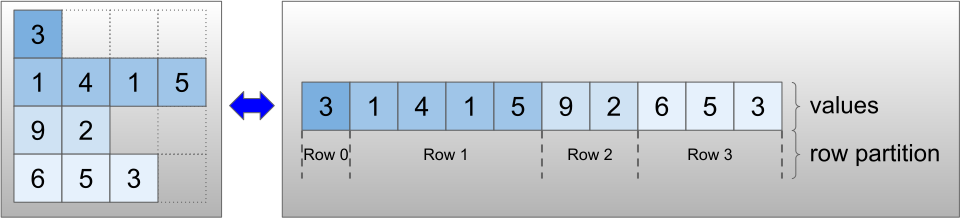The `row_partition` can be stored using four different encodings:

• `row_splits` is an integer vector specifying the split points between rows.
• `value_rowids` is an integer vector specifying the row index for each value.
• `row_lengths` is an integer vector specifying the length of each row.
• `uniform_row_length` is an integer scalar specifying a single length for all rows.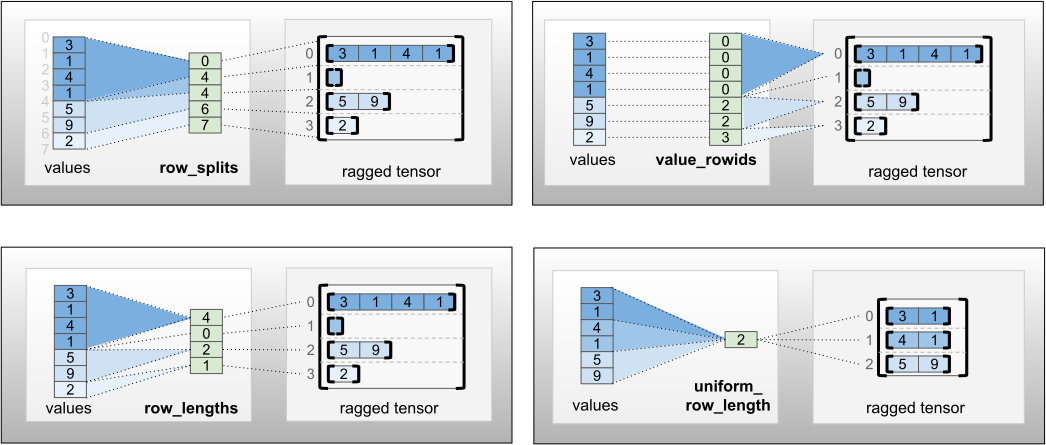An integer scalar `nrows` can also be included in the `row_partition` encoding to account for empty trailing rows with `value_rowids` or empty rows with `uniform_row_length`.

``````rt = tf.RaggedTensor.from_row_splits(
values=[3, 1, 4, 1, 5, 9, 2],
row_splits=[0, 4, 4, 6, 7])
print(rt)
``````
```<tf.RaggedTensor [[3, 1, 4, 1], [], [5, 9], ]>
```

The choice of which encoding to use for row partitions is managed internally by ragged tensors to improve efficiency in some contexts. In particular, some of the advantages and disadvantages of the different row-partitioning schemes are:

• Efficient indexing: The `row_splits` encoding enables constant-time indexing and slicing into ragged tensors.
• Efficient concatenation: The `row_lengths` encoding is more efficient when concatenating ragged tensors, since row lengths do not change when two tensors are concatenated together.
• Small encoding size: The `value_rowids` encoding is more efficient when storing ragged tensors that have a large number of empty rows, since the size of the tensor depends only on the total number of values. On the other hand, the `row_splits` and `row_lengths` encodings are more efficient when storing ragged tensors with longer rows, since they require only one scalar value for each row.
• Compatibility: The `value_rowids` scheme matches the segmentation format used by operations, such as `tf.segment_sum`. The `row_limits` scheme matches the format used by ops such as `tf.sequence_mask`.
• Uniform dimensions: As discussed below, the `uniform_row_length` encoding is used to encode ragged tensors with uniform dimensions.

### Multiple ragged dimensions

A ragged tensor with multiple ragged dimensions is encoded by using a nested `RaggedTensor` for the `values` tensor. Each nested `RaggedTensor` adds a single ragged dimension.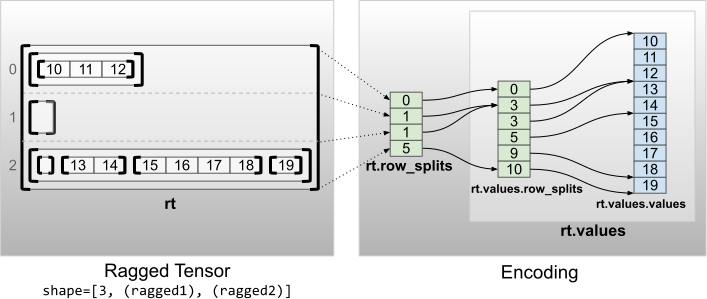``````rt = tf.RaggedTensor.from_row_splits(
values=tf.RaggedTensor.from_row_splits(
values=[10, 11, 12, 13, 14, 15, 16, 17, 18, 19],
row_splits=[0, 3, 3, 5, 9, 10]),
row_splits=[0, 1, 1, 5])
print(rt)
print("Shape: {}".format(rt.shape))
print("Number of partitioned dimensions: {}".format(rt.ragged_rank))
``````
```<tf.RaggedTensor [[[10, 11, 12]], [], [[], [13, 14], [15, 16, 17, 18], ]]>
Shape: (3, None, None)
Number of partitioned dimensions: 2
```

The factory function `tf.RaggedTensor.from_nested_row_splits` may be used to construct a RaggedTensor with multiple ragged dimensions directly by providing a list of `row_splits` tensors:

``````rt = tf.RaggedTensor.from_nested_row_splits(
flat_values=[10, 11, 12, 13, 14, 15, 16, 17, 18, 19],
nested_row_splits=([0, 1, 1, 5], [0, 3, 3, 5, 9, 10]))
print(rt)
``````
```<tf.RaggedTensor [[[10, 11, 12]], [], [[], [13, 14], [15, 16, 17, 18], ]]>
```

### Ragged rank and flat values

A ragged tensor's ragged rank is the number of times that the underlying `values` tensor has been partitioned (i.e. the nesting depth of `RaggedTensor` objects). The innermost `values` tensor is known as its flat_values. In the following example, `conversations` has ragged_rank=3, and its `flat_values` is a 1D `Tensor` with 24 strings:

``````# shape = [batch, (paragraph), (sentence), (word)]
conversations = tf.ragged.constant(
[[[["I", "like", "ragged", "tensors."]],
[["Oh", "yeah?"], ["What", "can", "you", "use", "them", "for?"]],
[["Processing", "variable", "length", "data!"]]],
[[["I", "like", "cheese."], ["Do", "you?"]],
[["Yes."], ["I", "do."]]]])
conversations.shape
``````
```TensorShape([2, None, None, None])
```
``````assert conversations.ragged_rank == len(conversations.nested_row_splits)
conversations.ragged_rank  # Number of partitioned dimensions.
``````
```3
```
``````conversations.flat_values.numpy()
``````
```array([b'I', b'like', b'ragged', b'tensors.', b'Oh', b'yeah?', b'What',
b'can', b'you', b'use', b'them', b'for?', b'Processing',
b'variable', b'length', b'data!', b'I', b'like', b'cheese.', b'Do',
b'you?', b'Yes.', b'I', b'do.'], dtype=object)
```

### Uniform inner dimensions

Ragged tensors with uniform inner dimensions are encoded by using a multidimensional `tf.Tensor` for the flat_values (i.e., the innermost `values`).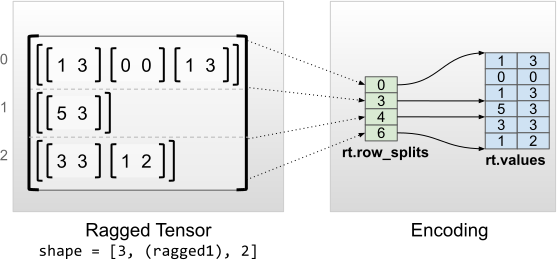``````rt = tf.RaggedTensor.from_row_splits(
values=[[1, 3], [0, 0], [1, 3], [5, 3], [3, 3], [1, 2]],
row_splits=[0, 3, 4, 6])
print(rt)
print("Shape: {}".format(rt.shape))
print("Number of partitioned dimensions: {}".format(rt.ragged_rank))
print("Flat values shape: {}".format(rt.flat_values.shape))
print("Flat values:\n{}".format(rt.flat_values))
``````
```<tf.RaggedTensor [[[1, 3],
[0, 0],
[1, 3]], [[5, 3]], [[3, 3],
[1, 2]]]>
Shape: (3, None, 2)
Number of partitioned dimensions: 1
Flat values shape: (6, 2)
Flat values:
[[1 3]
[0 0]
[1 3]
[5 3]
[3 3]
[1 2]]
```

### Uniform non-inner dimensions

Ragged tensors with uniform non-inner dimensions are encoded by partitioning rows with `uniform_row_length`.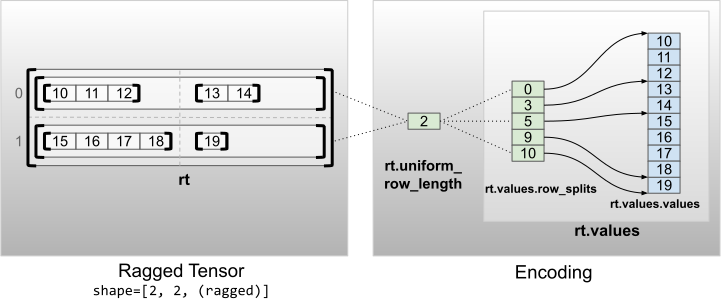``````rt = tf.RaggedTensor.from_uniform_row_length(
values=tf.RaggedTensor.from_row_splits(
values=[10, 11, 12, 13, 14, 15, 16, 17, 18, 19],
row_splits=[0, 3, 5, 9, 10]),
uniform_row_length=2)
print(rt)
print("Shape: {}".format(rt.shape))
print("Number of partitioned dimensions: {}".format(rt.ragged_rank))
``````
```<tf.RaggedTensor [[[10, 11, 12], [13, 14]],
[[15, 16, 17, 18], ]]>
Shape: (2, 2, None)
Number of partitioned dimensions: 2
```
[{ "type": "thumb-down", "id": "missingTheInformationINeed", "label":"Missing the information I need" },{ "type": "thumb-down", "id": "tooComplicatedTooManySteps", "label":"Too complicated / too many steps" },{ "type": "thumb-down", "id": "outOfDate", "label":"Out of date" },{ "type": "thumb-down", "id": "samplesCodeIssue", "label":"Samples / code issue" },{ "type": "thumb-down", "id": "otherDown", "label":"Other" }]
[{ "type": "thumb-up", "id": "easyToUnderstand", "label":"Easy to understand" },{ "type": "thumb-up", "id": "solvedMyProblem", "label":"Solved my problem" },{ "type": "thumb-up", "id": "otherUp", "label":"Other" }]